# Grammar, context-free

Jump to: navigation, search

CF-grammar

A context-sensitive grammar (cf. Grammar, context-sensitive) all rules of which have the form, whereis a non-terminal symbol andis a non-empty string (the so-called context-free rules). Languages generated by such grammars are said to be context-free languages. Thus, the language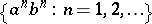is generated by the context-free grammar with rules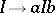,, whereis an initial symbol.

The condition thatbe non-empty may be omitted from the definition of a context-free language without materially altering the class of languages thereby (the only additional languages are those obtained from context-free languages by addition of the empty string). Context-free grammars constitute the class of formal grammars (cf. Grammar, formal) which is most frequently employed in applications; they are extensively used in the construction of mathematical models of natural languages (cf. Mathematical linguistics) and in the description of programming languages. The class of context-free languages is a proper subclass of the class of context-sensitive languages (e.g. the context-sensitive language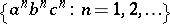is not context-free, cf. Grammar, context-sensitive) and coincides with the class of languages accepted by so-called push-down automata.

Each context-free grammar can be transformed, in an equivalent way, into Chomsky normal form — a context-free grammar all rules of which have the form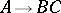and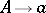, and also into Greibach normal form, all rules of which have the form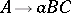,and(in both cases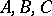are non-terminal symbols, whileis a terminal symbol). Context-free languages are also defined by categorial grammars, dominating grammars and dependency grammars (cf. Grammar, categorial; Grammar, dominating). Context-free languages are also sometimes defined by so-called normal systems of equations in languages, which are another form of notation of context-free grammars. The class of context-free languages is closed with respect to union, concatenation, permutation, and truncated iteration (and, in the presence of rules with an empty right-hand side, also with respect to iteration), and are not closed under intersection and complementation. A context-free grammaris called unambiguous if for each string of the languagethere is a unique derivation tree in. A context-free language is called unambiguous if it is generated by some unambiguous context-free grammar; otherwise it is called ambiguous (or inherently ambiguous). The following is an example of an ambiguous context-free language:If, for any context-free grammar generating a context-free languageand for any natural number, it is possible to find a string inwith more thanderivation trees in the given grammar, thenis said to have infinite degree of ambiguousness; an example is the language, where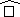indicates reversal (i.e. if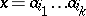, then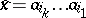).

A context-free language is said to be deterministic if it is accepted by some deterministic push-down automaton. Any deterministic language is unambiguous, but the converse is not true; for example, the unambiguous language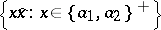is not deterministic.

## Complexity of derivation.

For any context-free grammar, the time complexity (number of elementary derivation steps) and the space complexity (maximal length intermediate phrase in derivation) of a derivation (cf. Grammar, generative) are bounded by linear functions from above and from below. For this reason other specific characteristics of the complexity of derivation are also introduced in the classification of context-free grammars. These characteristics are of two types: some of them ( "tree-like" characteristics) are constructed by representing the result in the form of a tree (cf. Grammar, context-sensitive), others ( "chain-like" characteristics) are based on the number or the distribution of the occurrences of non-terminal symbols into the individual steps of the derivation; in a number of important cases the "tree-like" characteristics may be connected to the "chain-like" characteristics with the same order of growth. Of the "tree-like" characteristics, the finest classification is given by the thickness, which is defined as follows. 1) To each vertexof a finite rooted tree is assigned a density of, a number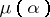, such that: ifis a terminal vertex, then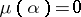; if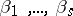are all the vertices entered into by arcs from, if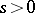and if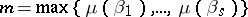, then: a) ifonly for one, then; b) otherwise. 2) The thickness of the root of the tree is said to be the thickness of the tree. 3) Ifis a context-free grammar, its thickness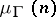is defined similarly to the time complexity, replacing the length of the derivation by the thickness of the derivation tree. The same order of growth as the thickness (for a context-free grammar) is displayed by the active capacity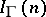, which is definable similarly to the capacity, the length of the stringbeing replaced by the number of occurrences of the non-terminal symbols in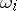. The thickness of all context-free grammars is bounded from above by a logarithmic function. There exist context-free languages for which the thicknesses of arbitrary context-free grammars by which they are generated have logarithmic order of growth, such as the set of all possible "regular sequences of parentheses" (i.e. sequences of left and right round brackets distributed in a manner which is accepted in mathematical expressions). At the same time the languages generated by context-free grammars whose thickness is bounded by constants constitute an extensive class, coinciding with the closure of the class of linear languages (cf. Grammar, linear) with respect to permutations. There exist infinite sequences of functions with order of growth intermediate between constant and logarithmic and such that for any two successive terms of the sequence it is possible to find a context-free language for which the lowest-order thickness of its generating context-free grammar is comprised between these functions. Other characteristics of complexity of derivation in context-free grammars are also employed.

Another subject of intensive study in context-free grammars is controlling derivations. Many different concepts of controlling derivations in context-free grammars have been proposed (a large part of which also being applicable to wider classes of grammars). Thus, a matrix grammar is obtained if some set of finite sequences of grammatical rules — "matrices" — is specified and if the derivations which are considered admissible are those for which the sequence of applications of the rules can be subdivided into the matrices (i.e. the rules are applied by "groups" only). In an ordered grammar a partial order is specified on the set of rules, and it is decided to employ at each step only those for which none of the rules which precede them in the sequence are applicable to the string obtained at this moment. In a programmed grammar two sets of rules are juxtaposed with each rule: "successful" and "unsuccessful" sets, and each rule is applied in two stages. The first stage consists of a check as to whether or not the left-hand side of the rule forms part of the string obtained at the moment; if it does, the second stage consists in replacing the left-hand side of the rule by its right-hand side and selecting the rule to be employed in the following step out of the "successful" set; if it does not, the second stage consists of the selection of the rule to be employed in the following stage out of the "unsuccessful" set. The class of languages generated by programmed context-free grammars without rules with an empty right-hand side is a proper subclass of the class of context-sensitive languages and contains the class of languages generated by context-free matrix grammars and ordered context-free grammars; both these classes are more extensive than the class of context-free languages. In the presence of rules with an empty right-hand side, programmed context-free grammars generate arbitrary recursively-enumerable languages.

## Algorithmic problems.

There exist algorithms by means of which it is possible to tell whether a language generated by some context-free grammar is empty or finite. However, the following properties of languages and relations between different languages are undecidable for the class of context-free grammars: whether the complement is empty, finite or context-free; whether the language is ambiguous, deterministic, linear, or regular;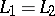;. There also exist context-free grammarsand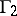for which there are no algorithms by which it is possible to recognize, for arbitrary stringsandin the basic alphabet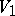of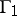(or in the basic alphabetof), whethercan be substituted forwith respect toand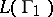(or whetherandare interchangeable with respect toand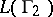; cf. Analytic model of a language).

## Complexity of recognition.

A string can be recognized as belonging to the language generated by a given context-free grammar with the aid of Cook's algorithm, which can be run on a Turing machine with one tape and one head, the duration of the run then being proportional to the fourth power of the string length (or to the third power if the number of tapes or heads is increased to three).

See also Grammar, regular; Grammar, linear.